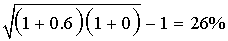#has-images #quantitative-methods-basic-concepts

#### Dollar-weighted and Time-weighted Rates of Return

The dollar-weighted rate of return is essentially the internal rate of return (IRR) on a portfolio. This approach considers the timing and amount of cash flows. It is affected by the timing of cash flows. If funds are added to a portfolio when the portfolio is performing well (poorly), the dollar-weighted rate of return will be inflated (depressed).

The time-weighted rate of return measures the compound growth rate of $1 initial investment over the measurement period. Time-weighted means that returns are averaged over time. This approach is not affected by the timing of cash flows; therefore, it is the preferred method of performance measurement. Example Jayson bought a share of IBM stock for$100 on December 31, 2000. On December 31, 2001, he bought another share for $150. On December 31, 2002, he sold both shares for$140 each. The stock paid a dividend of $10 per share at the end of each year. To calculate the dollar-weighted rate of return, you need to determine the timing and amount of cash flows for each year, and then set the present value of net cash flows to be 0: - 100 - 140/(1 + r) + 300/(1 + r)2 = 0. You can use the IRR function on a financial calculator to solve for r to get the dollar-weighted rate of return: r = 17%. To calculate the time-weighted rate of return: • Split the overall measurement period into equal sub-periods on the dates of cash flows. For the first year: • beginning price:$100
• dividends: $10 • ending price:$150

For the second year:

• beginning price: $300 (150 x 2) • dividends:$20 (10 x 2)
• ending price: \$280 (140 x 2)

• Calculate the holding period return (HPR) on the portfolio for each sub-period: HPR = (Dividends + Ending Price)/Beginning Price - 1. For the first year, HPR1: (150 + 10)/100 - 1 = 0.60. For the second year, HPR2: (280 + 20)/300 - 1 = 0.

• Calculate the time-weighted rate of return:

• If the measurement period < 1 year, compound holding period returns to get an annualized rate of return for the year.
• If the measurement period > 1 year, take the geometric mean of the annual returns.Learning Outcome Statements

d. calculate and compare the money-weighted and time-weighted rates of return of a portfolio and evaluate the performance of portfolios based on these measures;
If you want to change selection, open original toplevel document below and click on "Move attachment"